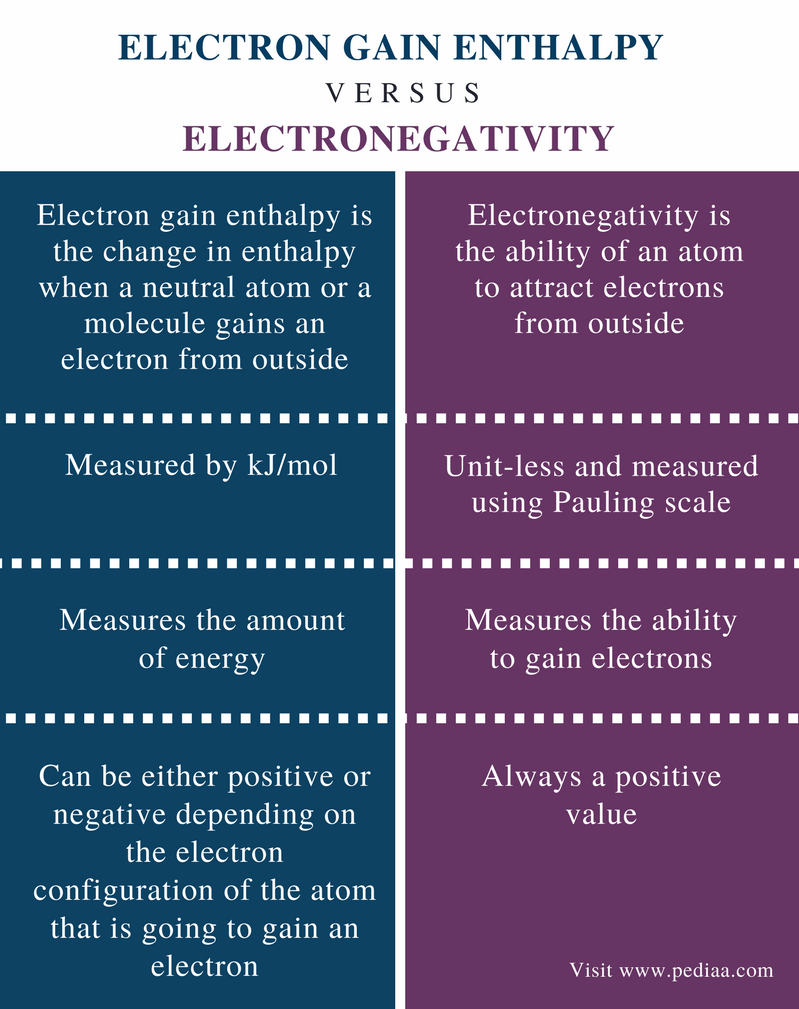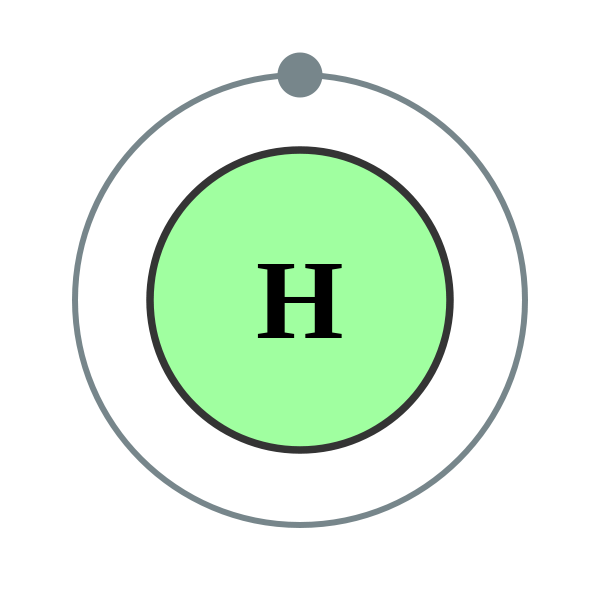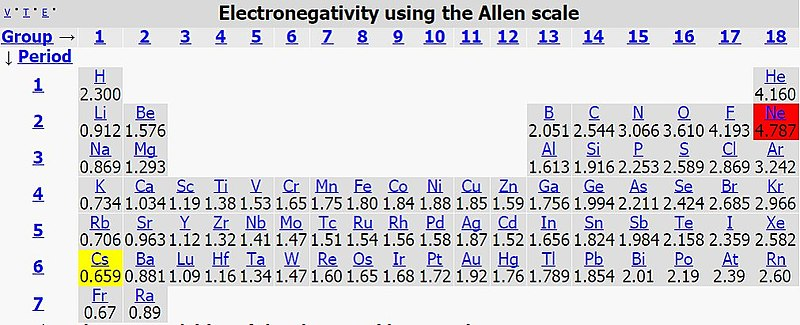# Difference Between Electron Gain Enthalpy and Electronegativity

## Main Difference – Electron Gain Enthalpy vs Electronegativity

An electron is a subatomic particle of an atom. Electrons are found everywhere since every matter is made up of atoms. However, electrons are very important in some chemical reactions since the exchange of electrons is the only difference between reactants and products in these reactions. Electron gain enthalpy and electronegativity are two chemical terms used to explain the binding of an electron with an atom. Electron gain enthalpy is the amount of energy released by an atom when an electron is gained from outside. Electronegativity is the ability of an atom to gain electrons from outside. Hence, electron gain enthalpy quantifies the electronegativity. The main difference between electron gain enthalpy and electronegativity is that electron gain enthalpy is measured by kJ/mol unit whereas electronegativity is unit-less and is measured by Pauling scale.

### Key Areas Covered

1. What is Electron Gain Enthalpy
– Definition, Units, Exothermic and Endothermic Reactions
2. What is Electronegativity
– Definition, Units of Measurement, Periodic Variations
3. What is the Difference Between Electron Gain Enthalpy and Electronegativity
– Comparison of Key Differences

Key Terms: Atom, Electron, Electron Affinity, Electronegativity, Electron Gain Enthalpy, Endothermic, Exothermic, Pauling Scale## What is Electron Gain Enthalpy

Electron gain enthalpy is the change in enthalpy when a neutral atom or a molecule gains an electron from outside. In other words, it is the amount of energy released when a neutral atom or molecule (in the gaseous phase) gains an electron from outside. Therefore, electron gain enthalpy is simply another term used for electron affinity. The unit for the measurement of electron gain enthalpy is kJ/mol.

The new electron addition causes the formation of a negatively charged chemical species. This can be represented by symbols as follows.

X       +       e       →     X      +       energy

However, there is a distinction between electron gain enthalpy and electron affinity. Electron gain enthalpy represents the energy released to the surrounding when an electron is gained whereas electron affinity represents the energy absorbed by the surrounding when an electron is gained. Therefore, electron gain enthalpy is a negative value while electron affinity is a positive value. Basically, both terms represent the same chemical process.Figure 1: Hydrogen electron configuration is 1s1. It can gain one more electron to fill its electron shell and become stable. Therefore, the electron gain enthalpy is a negative value for this electron gaining.

The electron gain enthalpy gives us an idea of how strong an electron is bound to an atom. Greater the amount of energy released, greater the electron gain enthalpy. The value of the electron gain enthalpy depends on the electron configuration of the atom to which an electron is gained. The addition of an electron to a neutral atom or a molecule releases energy. This is called an exothermic reaction. This reaction results in a negative ion. The electron gain enthalpy will be a negative value. But if another electron is going to be added to this negative ion, energy should be given in order to proceed with that reaction. This is because the incoming electron is repelled by the other electrons. This phenomenon is called an endothermic reaction. Here, the electron gain enthalpy will be a positive value.

## What is Electronegativity

Electronegativity is the ability of an atom to attract electrons from outside. This is a qualitative property of an atom, and in order to compare the electronegativity values of atoms in each element, a scale where relative electronegativity values reside is used. This scale is called “Pauling scale.” According to this scale, the highest electronegativity value that an atom can have is 4.0. The electronegativity values of other atoms are given a value considering their capabilities of attracting electrons.

Electronegativity depends on the atomic number and the size of the atom in an element. When considering the periodic table, Fluorine (F) is given the value 4.0 for its electronegativity since it is a small atom and the valence electrons are located near the nucleus. Thus, it can attract electrons from the outside easily. In addition, the atomic number of Fluorine is 9; it has a vacant orbital for one more electron in order to obey the octet rule. Therefore, Fluorine readily attracts electrons from outside.Figure 2: Allen scale is a different scale used to give the electronegativity of atoms. However, Pauling scale is the generally used scale in which 4.0 is the maximum electronegativity value.

Electronegativity causes a bond between two atoms to be polar. If one atom is more electronegative than the other atom, the atom with the higher electronegativity can attract electrons of the bond. This causes the other atom to have a partial positive charge due to lack of electrons around it. Therefore, electronegativity is the key to classify chemical bonds as polar covalent, nonpolar covalent, and ionic bonds. Ionic bonds occur between two atoms with a huge difference in electronegativity between them whereas covalent bonds occur between atoms with a slight difference in electronegativity between the atoms.

The electronegativity of elements varies periodically. The periodic table of elements has a better arrangement of elements according to their electronegativity values. When considering a period in the periodic table, the atomic size of each element decreases from left to right of the period. This is because the number of electrons present in the valence shell and the number of protons in the nucleus are increased, and thus, the attraction between electrons and the nucleus is increased gradually. Therefore, the electronegativity is also increased along the same period because the attraction that comes from the nucleus is increased. Then the atoms can easily attract electrons from the outside.

## Difference Between Electron Gain Enthalpy and Electronegativity

### Definition

Electron Gain Enthalpy: Electron gain enthalpy is the change in enthalpy when a neutral atom or a molecule gains an electron from outside.

Electronegativity: Electronegativity is the ability of an atom to attract electrons from outside.

### Unit of Measurement

Electron Gain Enthalpy: Electron gain enthalpy is measured by kJ/mol.

Electronegativity: Electronegativity is unit-less and is measured using Pauling scale.

### Measurement

Electron Gain Enthalpy: Electron gain enthalpy measures the amount of energy.

Electronegativity: Electronegativity measures the ability to gain electrons.

### Value

Electron Gain Enthalpy: Electron gain enthalpy can be either positive or negative depending on the electron configuration of the atom that is going to gain an electron.

Electronegativity: Electronegativity is always a positive value.

### Conclusion

Electron gain enthalpy measures the amount of energy released when an atom gains an electron from outside. Electronegativity measures the ability of an atom to gain an electron from outside. The main difference between electron gain enthalpy and electronegativity is that electron gain enthalpy is measured by kJ/mol unit whereas electronegativity is unit-less and is measured by Pauling scale.

##### Reference:

1. “Electron Gain Enthalpy – Chemistry, Class 11, Classification of Elements and Periodicity in Properties.” ClassNotes.org.in, 28 Mar. 2017, Available here.
2. “Electronegativity.” Chemistry LibreTexts, Libretexts, 29 Sept. 2017, Available here.

##### Image Courtesy:

1. “Electron shell 001 Hydrogen – no label” By commons:User:Pumbaa (original work by commons:User:Greg Robson)  (corresponding labeled version) (CC BY-SA 2.0 uk) via Commons Wikimedia
2. “Picture of allen electronegativity” By Mcardlep – (CC BY-SA 4.0)
via Commons Wikimedia